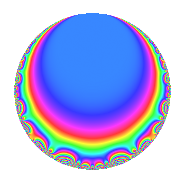# Properties

 Label 2563.1.b.dLevel 2563 Weight 1 Character orbit 2563.b Analytic conductor 1.279 Analytic rank 0 Dimension 2 Projective image $$S_{4}$$ CM/RM No Inner twists 2

# Related objects

## Newspace parameters

 Level: $$N$$ = $$2563 = 11 \cdot 233$$ Weight: $$k$$ = $$1$$ Character orbit: $$[\chi]$$ = 2563.b (of order $$2$$ and degree $$1$$)

## Newform invariants

 Self dual: No Analytic conductor: $$1.27910362738$$ Analytic rank: $$0$$ Dimension: $$2$$ Coefficient field: $$\Q(\sqrt{-2})$$ Coefficient ring: $$\Z[a_1, a_2]$$ Coefficient ring index: $$1$$ Projective image $$S_{4}$$ Projective field Galois closure of 4.2.2563.1 Artin image size $$48$$ Artin image $\GL(2,3)$ Artin field Galois closure of 8.2.16836267547.1

## $q$-expansion

Coefficients of the $$q$$-expansion are expressed in terms of $$\beta = \sqrt{-2}$$. We also show the integral $$q$$-expansion of the trace form.

 $$f(q)$$ $$=$$ $$q -\beta q^{2} + \beta q^{3} - q^{4} -\beta q^{5} + 2 q^{6} - q^{9} +O(q^{10})$$ $$q -\beta q^{2} + \beta q^{3} - q^{4} -\beta q^{5} + 2 q^{6} - q^{9} -2 q^{10} - q^{11} -\beta q^{12} + 2 q^{15} - q^{16} - q^{17} + \beta q^{18} -\beta q^{19} + \beta q^{20} + \beta q^{22} - q^{23} - q^{25} -\beta q^{29} -2 \beta q^{30} - q^{31} + \beta q^{32} -\beta q^{33} + \beta q^{34} + q^{36} + q^{37} -2 q^{38} - q^{41} + q^{43} + q^{44} + \beta q^{45} + \beta q^{46} + \beta q^{47} -\beta q^{48} + q^{49} + \beta q^{50} -\beta q^{51} -\beta q^{53} + \beta q^{55} + 2 q^{57} -2 q^{58} -2 q^{60} + \beta q^{62} + q^{64} -2 q^{66} + q^{68} -\beta q^{69} - q^{71} + q^{73} -\beta q^{74} -\beta q^{75} + \beta q^{76} - q^{79} + \beta q^{80} - q^{81} + \beta q^{82} + \beta q^{85} -\beta q^{86} + 2 q^{87} + q^{89} + 2 q^{90} + q^{92} -\beta q^{93} + 2 q^{94} -2 q^{95} -2 q^{96} -\beta q^{97} -\beta q^{98} + q^{99} +O(q^{100})$$ $$\operatorname{Tr}(f)(q)$$ $$=$$ $$2q - 2q^{4} + 4q^{6} - 2q^{9} + O(q^{10})$$ $$2q - 2q^{4} + 4q^{6} - 2q^{9} - 4q^{10} - 2q^{11} + 4q^{15} - 2q^{16} - 2q^{17} - 2q^{23} - 2q^{25} - 2q^{31} + 2q^{36} + 2q^{37} - 4q^{38} - 2q^{41} + 2q^{43} + 2q^{44} + 2q^{49} + 4q^{57} - 4q^{58} - 4q^{60} + 2q^{64} - 4q^{66} + 2q^{68} - 2q^{71} + 2q^{73} - 2q^{79} - 2q^{81} + 4q^{87} + 2q^{89} + 4q^{90} + 2q^{92} + 4q^{94} - 4q^{95} - 4q^{96} + 2q^{99} + O(q^{100})$$

## Character Values

We give the values of $$\chi$$ on generators for $$\left(\mathbb{Z}/2563\mathbb{Z}\right)^\times$$.

 $$n$$ $$1399$$ $$2333$$ $$\chi(n)$$ $$-1$$ $$-1$$

## Embeddings

For each embedding $$\iota_m$$ of the coefficient field, the values $$\iota_m(a_n)$$ are shown below.

For more information on an embedded modular form you can click on its label.

Label $$\iota_m(\nu)$$ $$a_{2}$$ $$a_{3}$$ $$a_{4}$$ $$a_{5}$$ $$a_{6}$$ $$a_{7}$$ $$a_{8}$$ $$a_{9}$$ $$a_{10}$$
2562.1
 1.41421i − 1.41421i
1.41421i 1.41421i −1.00000 1.41421i 2.00000 0 0 −1.00000 −2.00000
2562.2 1.41421i 1.41421i −1.00000 1.41421i 2.00000 0 0 −1.00000 −2.00000
 $$n$$: e.g. 2-40 or 990-1000 Significant digits: Format: Complex embeddings Normalized embeddings Satake parameters Satake angles

## Inner twists

Char. orbit Parity Mult. Self Twist Proved
1.a Even 1 trivial yes
2563.b Odd 1 yes

## Hecke kernels

This newform can be constructed as the intersection of the kernels of the following linear operators acting on $$S_{1}^{\mathrm{new}}(2563, [\chi])$$:

 $$T_{2}^{2} + 2$$ $$T_{17} + 1$$